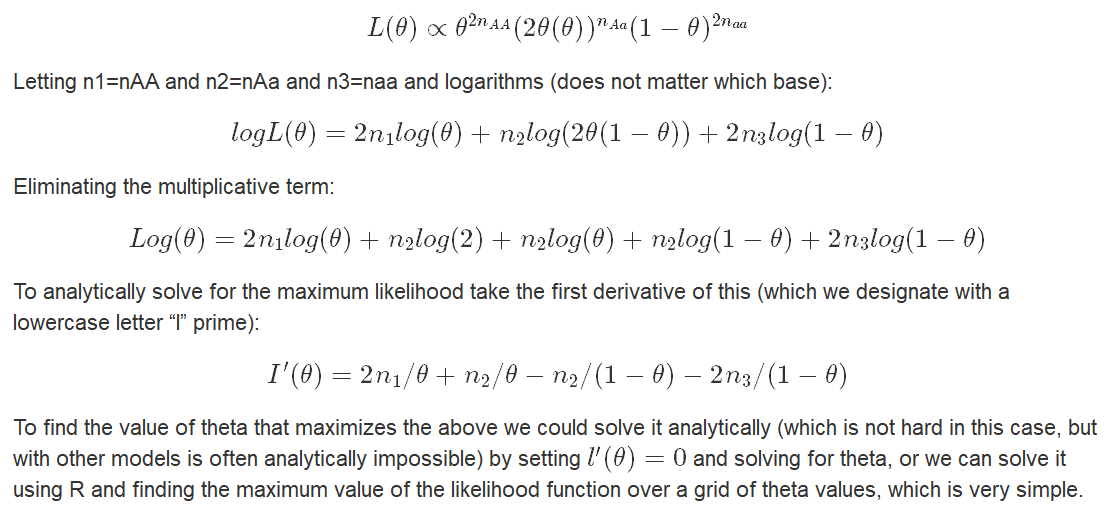The follow exercises will cover the concepts of:

• Random variables
• Parameters
• Discrete Distributions
• Continuous Distributions

The exercises will be solved using R. If you have any questions press red light of your monitor.

### Introduction

Every random variable has an associated probability distribution function. This function is called a probability mass function in the case of a discrete random variable or probability density function in the case of a continuous random variable. The distribution function is used to model how the outcome probabilities are associated with the values of the random variable.

For practical computations R has built-in-functions for the binomial, normal, t-Student, F, etc., where d stands for density, p for (cumulative) probability distribution, q for quantiles, and r for drawing random samples.

In https://stat.ethz.ch/R-manual/R-devel/library/stats/html/Distributions.html you can find the R functions for several distributions.

Bernoulli Distribution X ~ Ber(1,theta)

We call Bernoulli trial a single trial with two possible outcomes: success and failure, where theta is the probability of success.

Bernoulli distribution

Exercise 1 Let’s say it is known that 2.6% of the population has a certain genetic disease. Then when we randomly select one person and observe their disease status, we define X to be 1 if they have it and 0 if they don’t.

1.1 Using R, what is the probablity of the selected person has no disease.

``````dbinom(0,1,0.026)
``````

1.2 Plot the probability mass function (pmf) of this distribution

``````  prob<-c(dbinom(0,1,0.026),dbinom(1,1,0.026))
barplot(prob,ylab="P(X=k)",names.arg=c(0,1), width=1,xlim=c(0,4),ylim=c(0,1), main="Probability   mass function Ber(0.026)")
``````

Binomial Distribution X ~ Bin(n,theta)\$\$

The random variable which counts the number of successes in n independent and identical Bernoulli trials is called Binomial random variable.

The binomial distribution describes the behavior of a count variable X if the following conditions apply:

1. The number of events n is fixed.

2. Each observation is independent.

3. Each observation represents one of two outcomes (success or failure).

4. The probability of success theta is the same for each outcome.

Exercise 2. Suppose that for certain microRNA of size 20 the probability of a purine is binomially distributed with probability 0.7.

2.1. What is the probability of having 14 purines?

2.2 What is the probability of less than or equal to 14 purines?

2.3 What is the probability of strictly more than 10 purines?

2.4 How many purines do you expect? In other words: What is the mean of the distribution?

2.5 What is the standard deviation of the distribution?

``````#a)
dbinom(14,20,0.7)

#b)
pbinom(14,20,0.7)

#c)
1-pbinom(10,20,0.7)

#d)
mean_10<-20*0.7

#e)
sqrt(20*0.7*0.3)
``````

Exercise 3. What probability does this R code represent: pbinom(15,20,0.7)-pbinom(10,20,0.7)+dbinom(10,20,0.7)

P(10<=X<=20)

Normal Distribution X ~ N(mu,sigma)

where mu=E(X) and sigma=sqrt(var(X))

Normal distribution is a continuous distribution.

Exercise 4. The distribution of the expression values of the ALL patients on the Zyxin gene are distributed according to N(1.6; 0.42).

4.1 Compute the probability that the expression values are smaller than 1.2?

4.2 What is the probability that the expression values are between 1.2 and 2.0?

4.3 What is the 15th quantile of that distribution. What does it mean?

``````#a)
pnorm(1.2,1.6,0.42)

#b)
pnorm(2,1.6,0.42)-pnorm(1.2,1.6,0.42)

#c)
qnorm(0.15,1.6,0.42)
``````

All normal distributions can be converted into the standard normal curve by subtracting the mean and dividing by the standard deviation: Z=(X-mu)/sigma ~ N(0,1)

Such Z is called a standard normal random variable. The scale of Z has no units and it is called the standardized scale.

Exercise 5. Given a sample 0.12, 0.24, 0.01, 0.16, 0.18, 0.55,0.89, 1.00, 1.45 and 2.5 corresponding to intensity levels of one gene from 5 DNA chips.

Analyze the distribution considering normality using density function in R and construct a normal QQ-plot to check for normality. Apply a log2 transformation and repeat the analysis. Use the qqnorm() and qqline() functions to get the normal QQ-plot. Compare your results.

``````set<-c(0.12, 0.24, 0.01, 0.16, 0.18, 0.55,0.89, 1.00, 1.45,
2.5)

plot(density(set))

new_set<-log2(set)
plot(density(new_set))
qqnorm(set)
qqline(set)
qqnorm(new_set)
qqline(new_set)
``````

## Maximum Likelihood Estimation

Likelihood function is the function of the parameters given the data. The principle of maximum likelihood estimation is that somewhere on the range of parameter values, the likelihood function hits a maximum value. The parameter values for which the likelihood function obtains its maximum are called the maximum likelihood estimates for the parameters.

Exercise 6 A common use of maximum likelihood estimation in genetics is to estimate parameters for the Hardy Weinberg Equilibrium model for the distribution of genotypes in a population at equilibrium. In a given population gene pool, for a two allele gene locus, alleles A and a are at frequencies p and q, respectively. According to Hardy Weinberg equilibrium the genetic frequencies of genotypes are modeled by the simple equation: p^2+2pq+q^2=1.

AA Aa aa
p^2 2pq q^2

The likelihood for this follows a multinomial probability distribution that we can model, where parameter theta, theta=p (and therefore 1-theta=q, theta between 0 and 1).

6.1 Obtain the log likelihood function.6.2 Suppose we collect data from a population sample of 500 (assume the population obeys Hardy Weinberg equilibrium) and obtain the data in Table:

Genotype Data Variable
AA 134 n_1
Aa 266 n_2
aa 100 n_3

Program R to solve for the maximum likelihood estimate of theta given this data.

``````#Create a grid of theta values
theta<-1:1000/1000

#Create a data vector to store (log)likelihood values
lik<-vector(length=1000)

#Enter data
n1<-134
n2<-266
n3<-100

#Given data, evaluate log likelihood
for(i in 1:1000){
lik[i]<-2*n1*log(theta[i])+n2*log(2)+n2*log(theta[i])+
n2*log(1-theta[i])+2*n3*log(1-theta[i])}

# Use which function to determine max value of lik

which(lik==max(lik))

lik

#MLE value for theta (corresponding vector index to lik)
theta
``````

6.3 Plot the results

``````plot(theta,lik,xlab="theta",ylab="log likelihood",